Water Content Determination - Pycnometer Method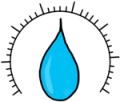Water content, which is also called moisture content, is the quantity of water contained in a material, such as soil.

Water content is denoted by small letter w.

Water content of any soil sample is defined as the ratio of weight of water to the weight of solids present in that sample. We also multiply it by hundred because it is usually expressed as percentage.Water content of soil is an important parameter which influences the behavior of soil.

Water content can be determined using several methods applied on small soil sample.

Oven drying method takes a long time in determining the water content, hence we need a quicker approach for the purpose.

So lets discuss the Pycnometer method

It is a quick laboratory method.

Pycnometer is a glass bottle with a conical cap which has a little hole at the top. Its capacity is approximately 900ml.

We start the experiment by taking the weight of the empty and completely dry pycnometer bottle with its cap. And we say this weight as W1.

Next, we take some 200 to 400 gm of moist soil into the bottle and weigh it again. And we say this weight as W2.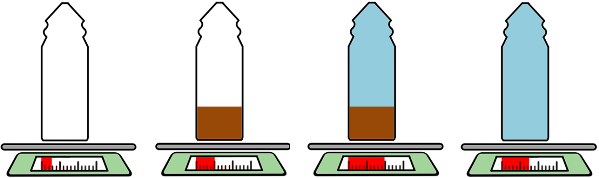Then pycnometer is filled with water completely upto the conical top and weighed again. This weight is W3.

Next, the bottle is emptied and cleaned thoroughly. Then it is filled upto the top only with water and weighed again. This weight is W4.

Now we have four weights…

W1 = weight of empty pycnometer

W2 = weight of pycnometer + moist soil sample

W3 = weight of pycnometer + moist soil sample + water

W4 = weight of pycnometer + water

We know water content as weight of water to the weights of solids.Our purpose here is to find weight of water and weights of solids to get the value of water content of soil.

From weight W2 if we remove weight of empty bottle which is W1 and weight of solids WS then we are left with weight of water in the soil sample.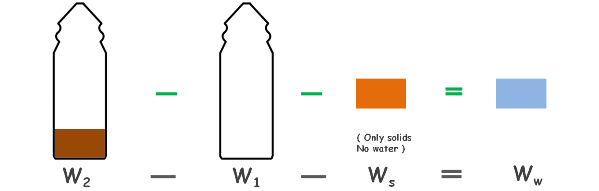Write it in the equation form.Now in the water content equation substitute this weight of water.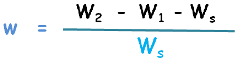Solving it a little we get…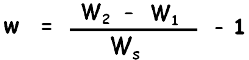.............(1)

Lets say this equation as equation number one. Now only weight of solids is unknown to get the water content.

Now in weight W3, if we replace all the solids with water then this weight will become w4.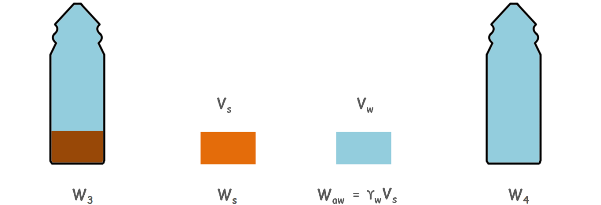We can see that volume of solids and volume of added water are same.

But they will weigh differently as they have different unit weights.

So weight of solids Ws and weight of water of the equivalent volume of solids, which will be unit weight of water multiplied by volume of water or volume of solids (as they are same)

Writing it in the equation form….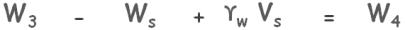We can see that volume of solids can be written as weight of solids divided by its unit weight and we can write unit weight of solids as specific gravity of solids multiplied by unit weight of water.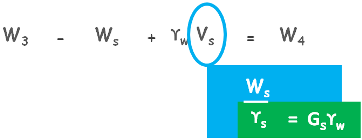After rearranging the equation we get the value of weight of solids.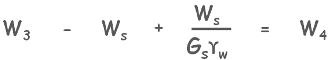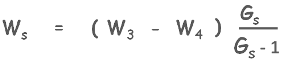substitute this value in the equation number one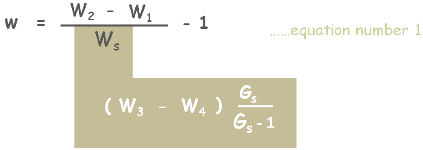After rearranging, we will get the water content we desired.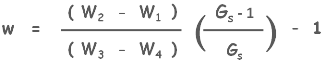We multiply it by 100 if we want water content as percentage.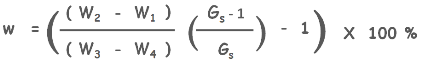This method is more suitable for the cohesionless soils as their particles do not stuck with each other very closely hence we do not face difficulty in removing the entrapped air.

Tags : pycnometer, soil test

Published on :2019-09-06